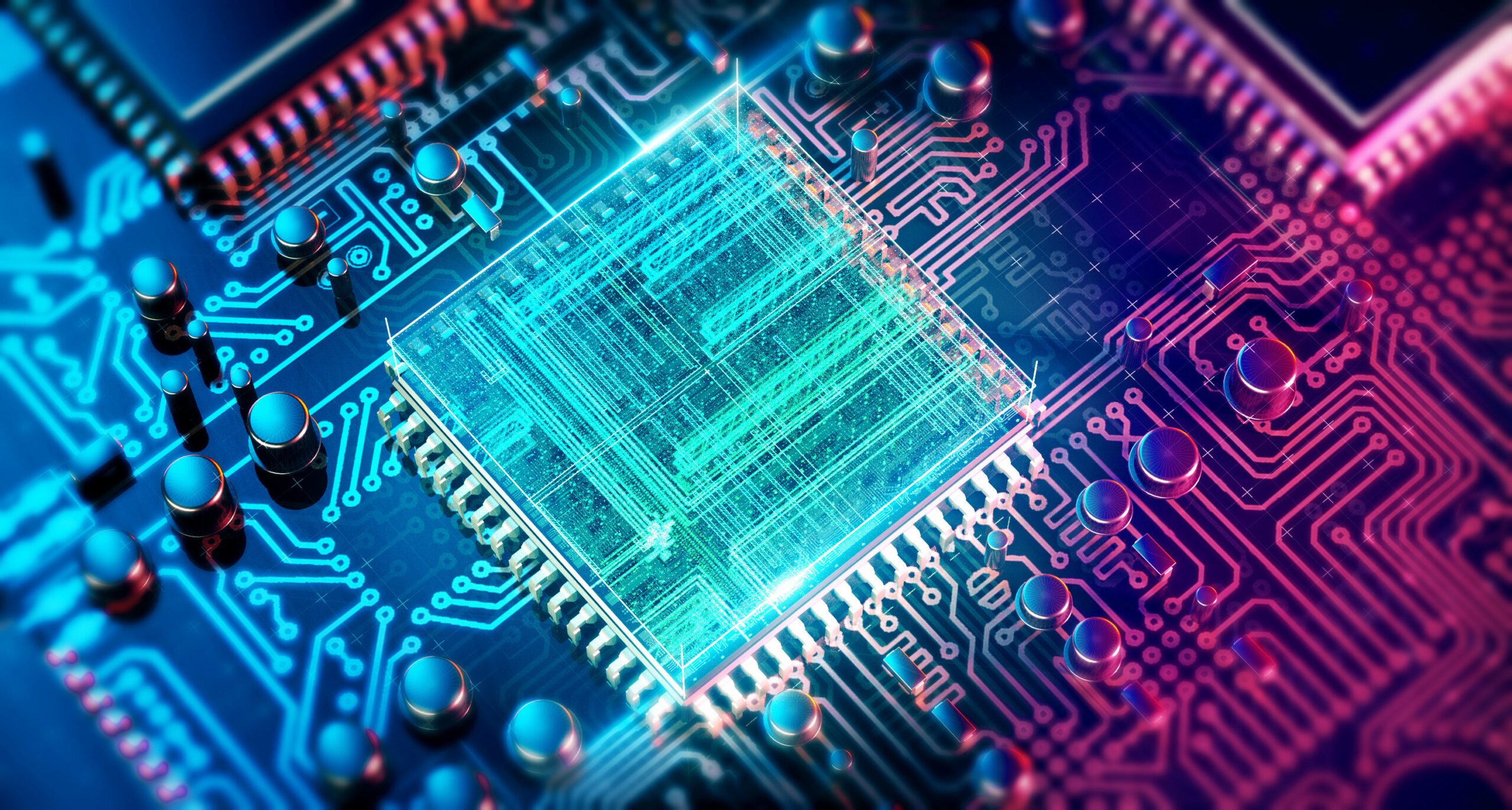In the classical computation model, the computer has access to memory which can be found in one of a finite set of possible states, each of which is physically distinct. The state of this memory is generally represented as a string of symbols 0 and 1. In the classical computation model, the fundamental unit of memory is called bit and the size of the memory can be measured in terms of the number of bits required to represent fully the state of the memory.  If the memory obeys laws of quantum physics, the state of memory could be found in a superposition of the possible bit strings and these strings could be found entangled with other bit strings. In the quantum computation model, the fundamental unit of memory is called a qubit which gives quantum computers its superior computing powers. In classical computers, two bits represent only two bits of information, however, in quantum computers, two qubits represent four bits of information. In quantum computers, N qubits represent 2N classical bits of information, whereas, in classical computers, N bits represent only N bits of information. Therefore, quantum computers could arrive at a result more efficiently than classical computers.Figure 1: Flow chart of information processed in computers

Figure 1 represents a flow chart of information processed in computers. A circuit chip comprises of basic modules which contain logic gates which contain transistors. A transistor is a fundamental data processor that behaves like a switch that controls the flow of information. I discussed the working of transistors in much detail in my previous blog post. The flow of information in transistors could be represented as a string of symbols that I discussed earlier. More complex information could be represented in more number of bits. Many transistors combine to form logic gates that combine with other logic gates to form basic modules. These modules process mathematical operations like addition, subtraction, multiplication, division, etc. With many such modules, we can process any data in computers. However, as I discussed in my previous blog post about the reduction in the size of transistors every two years, soon a limit will be reached where a classical transistor will be useless as quantum effects will take over.

The charge carriers in transistors will tunnel across the p-n junction through a phenomenon called quantum tunneling. Thus, to solve this problem scientists across the globe are building and studying quantum computers.  These quantum computers use qubit which could be in a superposition state of possible bite strings and could be entangled with other qubits. Unlike classical logic gates which get a classical set of inputs and produce a definite classical output, a quantum gate could manipulate input of superposition, rotate probabilities and produce another superposition at its output.  Many quantum gates could be combined to form a quantum module that sets up some qubits, applies quantum gates and measures the outcome which collapses superposition into classical bits. These classical bits could then be used to process mathematical operations like addition, subtraction, multiplication, division, etc.  Thus, allowing scientists to explore the applications of quantum computers.

Cryptography is one of the important fields of applications of quantum computers. Unlike classical computers which can take N years to find prime factors of a number, a quantum computer could take N1/2 years to find the same. Thus making quantum computers the most efficient way to encrypt the private key in cryptography. Another application of quantum computers is simulating quantum phenomena which would be way more efficient than simulating in classical computers. This could revolutionize the field of physics, chemistry, medicine, etc. With such amazing implications, quantum computer faces lots of challenges like maintaining extreme cold temperatures, removing quantum decoherence, etc. Thus, making quantum computers inconvenient to use in day to day life.

You must be logged in to post a comment.